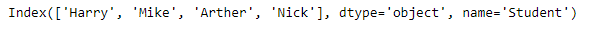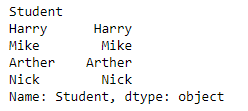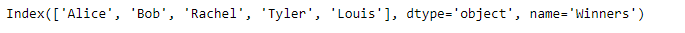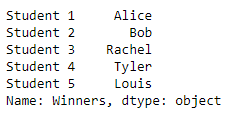Related Articles

# Python | Pandas Index.to_series()

• Last Updated : 24 Dec, 2018

Python is a great language for doing data analysis, primarily because of the fantastic ecosystem of data-centric python packages. Pandas is one of those packages and makes importing and analyzing data much easier.

Pandas` Index.to_series()` function create a Series with both index and values equal to the index keys useful with map for returning an indexer based on an index. It is possible to set a new index label for the newly created Series by passing the list of new index labels.

Syntax: Index.to_series(index=None, name=None)

Parameters :
index : index of resulting Series. If None, defaults to original index
name : name of resulting Series. If None, defaults to name of original index

Returns : Series : dtype will be based on the type of the Index values.

Example #1: Use `Index.to_series()` function to convert the index into a Series.

 `# importing pandas as pd``import` `pandas as pd`` ` `# Creating the index``idx ``=` `pd.Index([``'Harry'``, ``'Mike'``, ``'Arther'``, ``'Nick'``],``                                   ``name ``=``'Student'``)`` ` `# Print the Index``print``(idx)`

Output :Let’s convert the index into a Series.

 `# convert the index into a series``idx.to_series()`

Output :The function has converted the index into a series. By default, the function has created the index of the series using the values of the original Index.

Example #2: Use `Index.to_series()` function to convert the index into a series such that the series created uses new index value.

 `# importing pandas as pd``import` `pandas as pd`` ` `# Creating the index``idx ``=` `pd.Index([``'Alice'``, ``'Bob'``, ``'Rachel'``, ``'Tyler'``, ``'Louis'``],``                                            ``name ``=``'Winners'``)`` ` `# Print the Index``print``(idx)`

Output :Let’s convert the index into a series.

 `# convert the index into a series``idx.to_series(index ``=``[``'Student 1'``, ``'Student 2'``, ``'Student 3'``,``                                  ``'Student 4'``, ``'Student 5'``])`

Output :The function has converted the index into a series. We have passed a list of index labels to be used for the newly created Series.

Attention geek! Strengthen your foundations with the Python Programming Foundation Course and learn the basics.

To begin with, your interview preparations Enhance your Data Structures concepts with the Python DS Course. And to begin with your Machine Learning Journey, join the Machine Learning – Basic Level Course

My Personal Notes arrow_drop_up